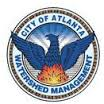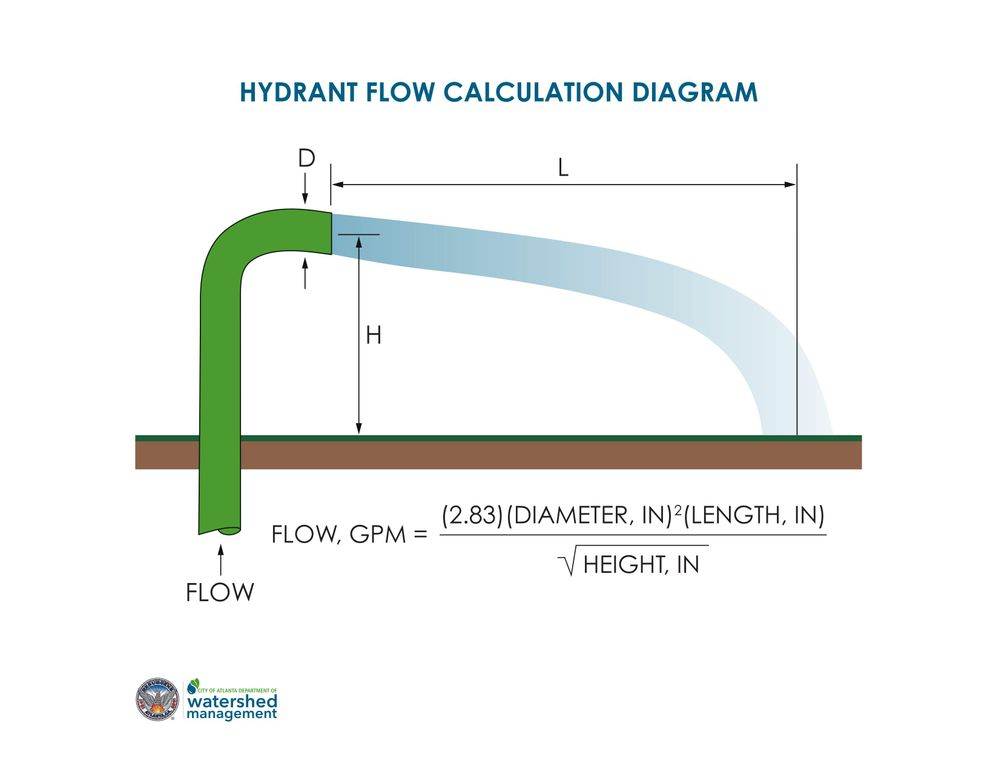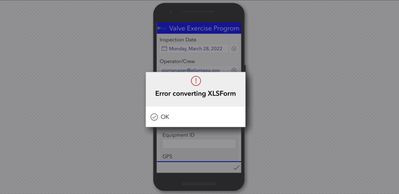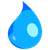# Fire Hydrant Flushing Formula

474
4
03-28-2022 06:02 AMOccasional Contributor

Need assistance creating the following formula to capture GPM from fire hydrant. Attached is the xlsx form1 Solution

Accepted SolutionsOccasional Contributor

Formula to calculate Gallons per Minute for a hydrant flush

round(((2.83*(pow(\${outlet_size},2)*\${water_length})) div (sqrt(\${height})))*\${time_flow},2

4 RepliesbyEsri Notable Contributor

((2.83*(pow(\${outlet_size},2)*\${water_length})) div (sqrt(\${height}))

or

round(((2.83*(pow(\${outlet_size},2)*\${water_length})) div (sqrt(\${height})),2)Occasional Contributor

I get an error message when using both formulas

.Occasional Contributor

Formula to calculate Gallons per Minute for a hydrant flush

round(((2.83*(pow(\${outlet_size},2)*\${water_length})) div (sqrt(\${height})))*\${time_flow},2byEsri Contributor

Cross-posting due similar topics related to fire-hydrant flow estimates.

https://community.esri.com/t5/arcgis-survey123-questions/calculation-for-hydrant-flow-rate/m-p/11645...

Given that the flow leaving a hydrant is turbulent rather than laminar, it could be anticipated that there would be substantial variation in the calculation results per site.  Not to mention determining/deciding where to actually measure the L when a hydrant spray/spread hits the ground could vary based on observer methods.  If such an approximated result aligns with the data needing to be recorded, a timed flow x estimated flowrate may provide a rapid and standardized approximation (simple calculation).  If accuracy is required for the data entry, a temporary flow meter would be ideal.